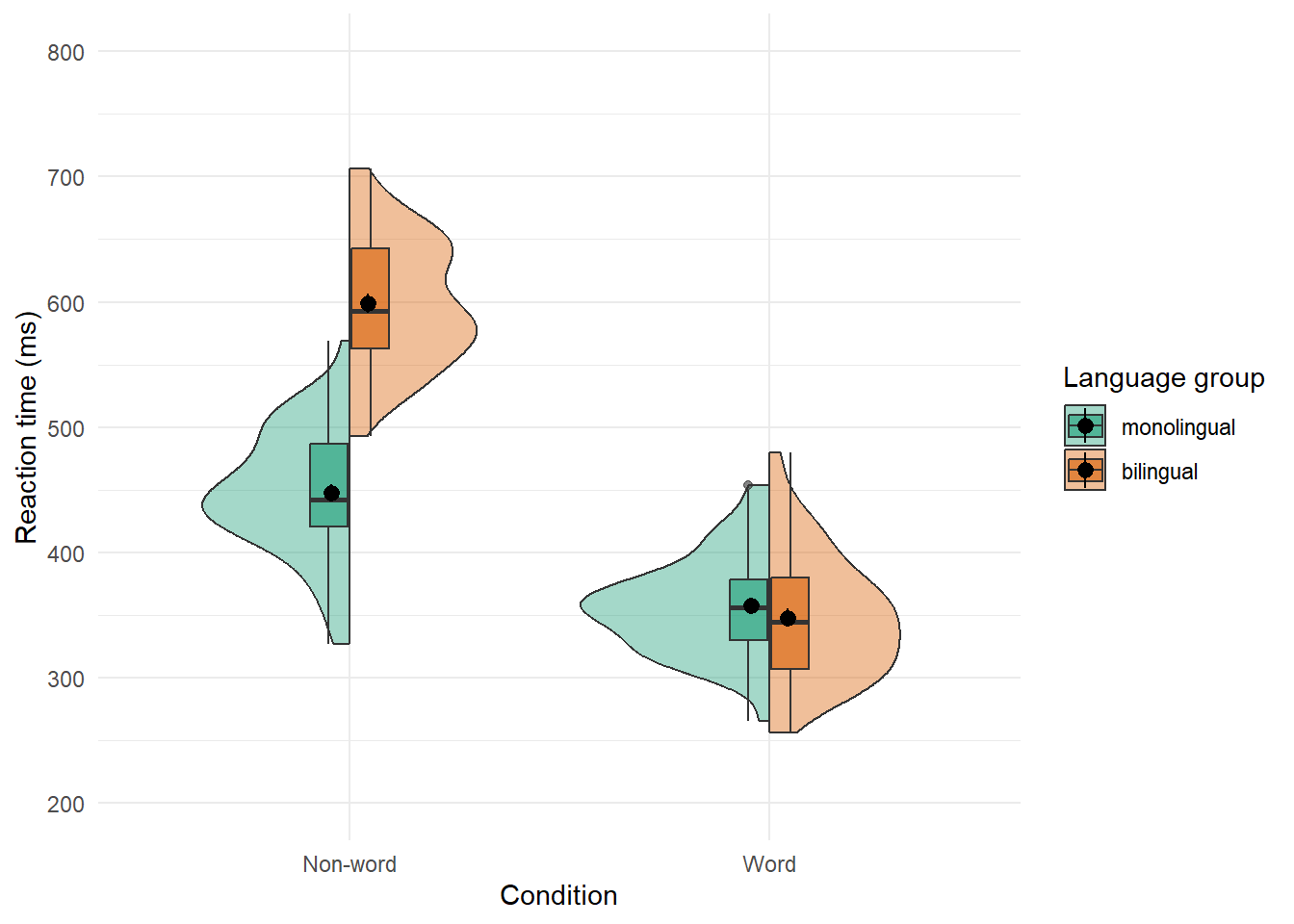# 4 Section 2

For the second session, we're going to do a whistle-stop tour through the following paper:

Nordmann, E., McAleer, P., Toivo, W., Paterson, H. & DeBruine, L. (accepted). Data visualisation using R, for researchers who don't use R. Advances in Methods and Practices in Psychological Science.

The first half of the session will consolidate the skills and functions you learned in Session 1 with a bit of extra data wrangling, however, we'll use simulated experimental data so that you can start thinking about how you might plot types of data you're likely to encounter in research. The second half will present some more advanced plots. Please note that there is extra detail and context in the paper that may not be covered in this workshop so we'd encourage reading the full paper if you haven't already.

## 4.1 Set-up

Open your Workshop project and do the following:

• Create and save a new R Markdown document named Session 2. get rid of the default template text from line 11 onwards.
• In your project folder, create a new sub-folder named `data`.
• Download the simulated dataset from the Open Science Framework and save it in your newly created data folder.
• Add the below code to the set-up chunk and then run the code to load the packages and data.
``````library(tidyverse)
library(patchwork)

## 4.2 Simulated dataset

For the purpose of this tutorial, we will use simulated data for a 2 x 2 mixed-design lexical decision task in which 100 participants must decide whether a presented word is a real word or a non-word. There are 100 rows (1 for each participant) and 7 variables:

• Participant information:

• `id`: Participant ID
• `age`: Age
• 1 between-subject independent variable (IV):

• `language`: Language group (1 = monolingual, 2 = bilingual)
• 4 columns for the 2 dependent variables (DVs) of RT and accuracy, crossed by the within-subject IV of condition:

• `rt_word`: Reaction time (ms) for word trials
• `rt_nonword`: Reaction time (ms) for non-word trials
• `acc_word`: Accuracy for word trials
• `acc_nonword`: Accuracy for non-word trials

## 4.3 Checking and cleaning your data

You should always check after importing data that the resulting table looks like you expect. To view the dataset, click `dat` in the environment pane or run `View(dat)` in the console. The environment pane also tells us that the object `dat` has 100 observations of 7 variables, and this is a useful quick check to ensure one has loaded the right data. Note that the 7 variables have an additional piece of information `chr` and `num`; this specifies the kind of data in the column. Similar to Excel and SPSS, R uses this information (or variable type) to specify allowable manipulations of data. For instance character data such as the `id` cannot be averaged, while it is possible to do this with numerical data such as the `age`.

### 4.3.1 Handling numeric factors

Another useful check is to use the functions `summary()` and `str()` (structure) to check what kind of data R thinks is in each column. Run the below code and look at the output of each, comparing it with what you know about the simulated dataset:

``````summary(dat)
str(dat)``````

Because the factor `language` is coded as 1 and 2, R has categorised this column as containing numeric information and unless we correct it, this will cause problems for visualisation and analysis. The code below shows how to recode numeric codes into labels.

• `mutate()` makes new columns in a data table, or overwrites a column;
• `factor()` translates the language column into a factor with the labels "monolingual" and "bilingual". You can also use `factor()` to set the display order of a column that contains words. Otherwise, they will display in alphabetical order. In this case we are replacing the numeric data (1 and 2) in the `language` column with the equivalent English labels `monolingual` for 1 and `bilingual` for 2. At the same time we will change the column type to be a factor, which is how R defines categorical data.
``````dat <- mutate(dat, language = factor(
x = language, # column to translate
levels = c(1, 2), # values of the original data in preferred order
labels = c("monolingual", "bilingual") # labels for display
))``````

Make sure that you always check the output of any code that you run. If after running this code `language` is full of `NA` values, it means that you have run the code twice. The first time would have worked and transformed the values from `1` to `monolingual` and `2` to `bilingual`. If you run the code again on the same dataset, it will look for the values `1` and `2`, and because there are no longer any that match, it will return NA. If this happens, you will need to reload the dataset from the csv file.

A good way to avoid this is never to overwrite data, but to always store the output of code in new objects (e.g., `dat_recoded`) or new variables (`language_recoded`). For the purposes of this tutorial, overwriting provides a useful teachable moment so we'll leave it as it is.

## 4.4 Basic plot recap

The code for the next couple of plots should be quite familiar from session 1. Before you run the code, try to visualise what it will look like from reading the code.

A bar chart of counts for the number of participants:

``````ggplot(dat, aes(language)) +
geom_bar() +
scale_x_discrete(name = "Language group",
labels = c("Monolingual", "Bilingual")) +
scale_y_continuous(name = "Number of participants",
breaks = seq(from = 0, to = 50, by = 10))``````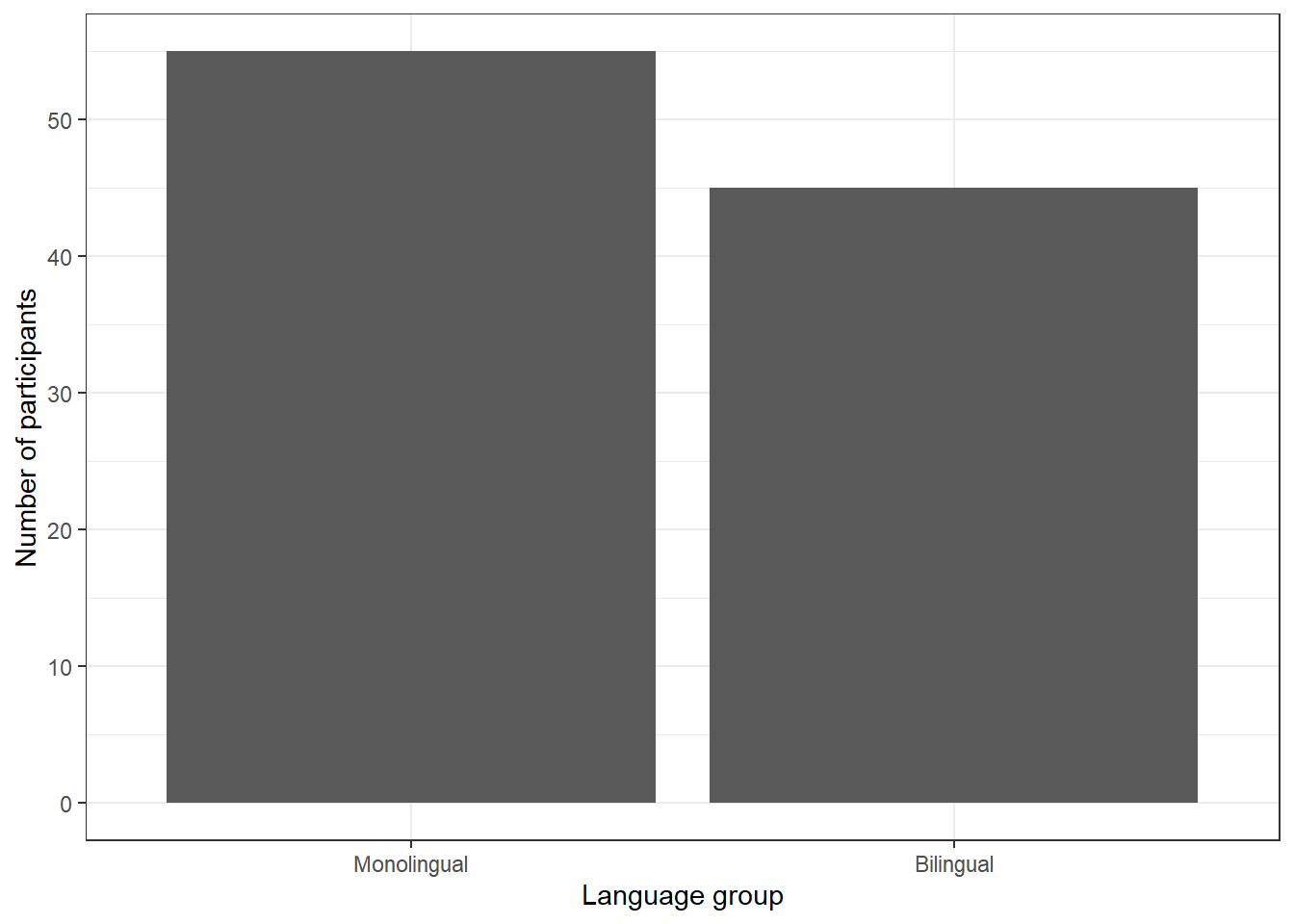Figure 4.1: Bar chart of counts.

A histogram of participant age:

``````ggplot(dat, aes(age)) +
geom_histogram(binwidth = 1, fill = "wheat", color = "black") +
scale_x_continuous(name = "Participant age (years)") +
theme_minimal()``````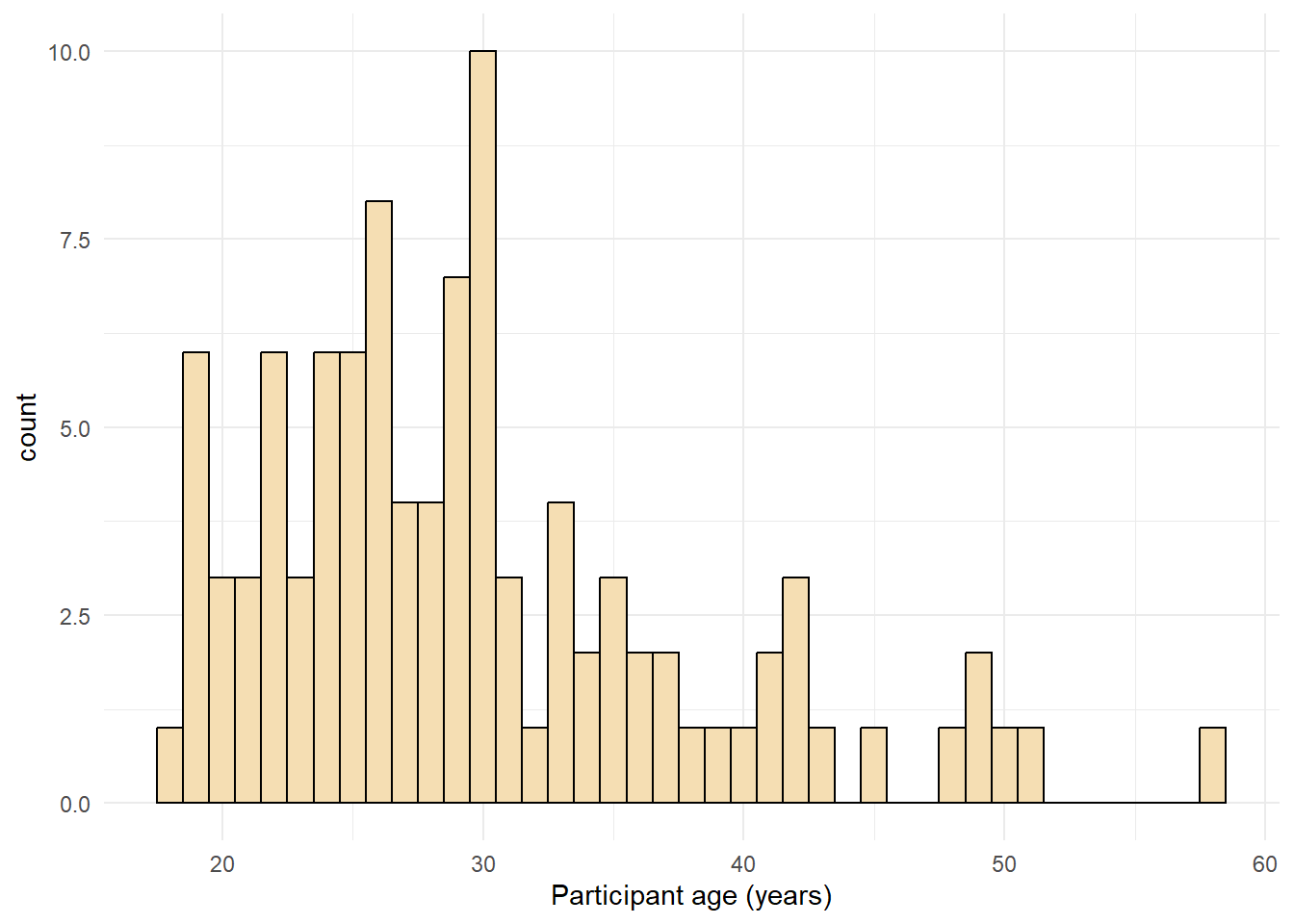Figure 4.2: Histogram with a custom theme.

## 4.5 Data formats

To visualise the experimental reaction time and accuracy data using `ggplot2`, we first need to reshape the data from wide format to long format. This step can cause friction with novice users of R. Traditionally, psychologists have been taught data skills using wide-format data. Wide-format data typically has one row of data for each participant, with separate columns for each score or variable. For repeated-measures variables, the dependent variable is split across different columns. For between-groups variables, a separate column is added to encode the group to which a participant or observation belongs.

The simulated lexical decision data is currently in wide format (see Table 4.1), where each participant's aggregated reaction time and accuracy for each level of the within-subject variable is split across multiple columns for the repeated factor of condition (words versus non-words).

Table 4.1: Data in wide format.
id age language rt_word rt_nonword acc_word acc_nonword
S001 22 monolingual 379.46 516.82 99 90
S002 33 monolingual 312.45 435.04 94 82
S003 23 monolingual 404.94 458.50 96 87
S004 28 monolingual 298.37 335.89 92 76
S005 26 monolingual 316.42 401.32 91 83
S006 29 monolingual 357.17 367.34 96 78

Moving from using wide-format to long-format datasets can require a conceptual shift on the part of the researcher and one that usually only comes with practice and repeated exposure. It may be helpful to make a note that “row = participant” (wide format) and “row = observation” (long format) until you get used to moving between the formats. For our example dataset, adhering to these rules for reshaping the data would produce Table 4.2. Rather than different observations of the same dependent variable being split across columns, there is now a single column for the DV reaction time, and a single column for the DV accuracy. Each participant now has multiple rows of data, one for each observation (i.e., for each participant there will be as many rows as there are levels of the within-subject IV). Although there is some repetition of age and language group, each row is unique when looking at the combination of measures.

Table 4.2: Data in the correct format for visualization.
id age language condition rt acc
S001 22 monolingual word 379.46 99
S001 22 monolingual nonword 516.82 90
S002 33 monolingual word 312.45 94
S002 33 monolingual nonword 435.04 82
S003 23 monolingual word 404.94 96
S003 23 monolingual nonword 458.50 87

The benefits and flexibility of this format will hopefully become apparent as we progress through the workshop, however, a useful rule of thumb when working with data in R for visualisation is that anything that shares an axis should probably be in the same column. For example, a simple boxplot showing reaction time by condition would display the variable `condition` on the x-axis with bars representing both the `word` and `nonword` data, and `rt` on the y-axis. Therefore, all the data relating to `condition` should be in one column, and all the data relating to `rt` should be in a separate single column, rather than being split like in wide-format data.

### 4.5.1 Wide to long format

We have chosen a 2 x 2 design with two DVs, as we anticipate that this is a design many researchers will be familiar with and may also have existing datasets with a similar structure. However, it is worth normalising that trial-and-error is part of the process of learning how to apply these functions to new datasets and structures. Data visualisation can be a useful way to scaffold learning these data transformations because they can provide a concrete visual check as to whether you have done what you intended to do with your data.

#### 4.5.1.1 Step 1: `pivot_longer()`

The first step is to use the function `pivot_longer()` to transform the data to long-form. We have purposefully used a more complex dataset with two DVs for this tutorial to aid researchers applying our code to their own datasets. Because of this, we will break down the steps involved to help show how the code works.

This first code ignores that the dataset has two DVs, a problem we will fix in step 2. The pivot functions can be easier to show than tell - you may find it a useful exercise to run the below code and compare the newly created object `long` (Table 4.3) with the original `dat` Table 4.1 before reading on.

``````long <- pivot_longer(data = dat,
cols = rt_word:acc_nonword,
names_to = "dv_condition",
values_to = "dv")``````
• As with the other tidyverse functions, the first argument specifies the dataset to use as the base, in this case `dat`. This argument name is often dropped in examples.

• `cols` specifies all the columns you want to transform. The easiest way to visualise this is to think about which columns would be the same in the new long-form dataset and which will change. If you refer back to Table 4.1, you can see that `id`, `age`, and `language` all remain, while the columns that contain the measurements of the DVs change. The colon notation `first_column:last_column` is used to select all variables from the first column specified to the last In our code, `cols` specifies that the columns we want to transform are `rt_word` to `acc_nonword`.

• `names_to` specifies the name of the new column that will be created. This column will contain the names of the selected existing columns.

• Finally, `values_to` names the new column that will contain the values in the selected columns. In this case we'll call it `dv`.

At this point you may find it helpful to go back and compare `dat` and `long` again to see how each argument matches up with the output of the table.

Table 4.3: Data in long format with mixed DVs.
id age language dv_condition dv
S001 22 monolingual rt_word 379.46
S001 22 monolingual rt_nonword 516.82
S001 22 monolingual acc_word 99.00
S001 22 monolingual acc_nonword 90.00
S002 33 monolingual rt_word 312.45
S002 33 monolingual rt_nonword 435.04

#### 4.5.1.2 Step 2: `pivot_longer()` adjusted

The problem with the above long-format data-set is that `dv_condition` combines two variables - it has information about the type of DV and the condition of the IV. To account for this, we include a new argument `names_sep` and adjust `name_to` to specify the creation of two new columns. Note that we are pivoting the same wide-format dataset `dat` as we did in step 1.

``````long2 <- pivot_longer(data = dat,
cols = rt_word:acc_nonword,
names_sep = "_",
names_to = c("dv_type", "condition"),
values_to = "dv")``````
• `names_sep` specifies how to split up the variable name in cases where it has multiple components. This is when taking care to name your variables consistently and meaningfully pays off. Because the word to the left of the separator (`_`) is always the DV type and the word to the right is always the condition of the within-subject IV, it is easy to automatically split the columns.

• Note that when specifying more than one column name, they must be combined using `c()` and be enclosed in their own quotation marks.

Table 4.4: Data in long format with dv type and condition in separate columns.
id age language dv_type condition dv
S001 22 monolingual rt word 379.46
S001 22 monolingual rt nonword 516.82
S001 22 monolingual acc word 99.00
S001 22 monolingual acc nonword 90.00
S002 33 monolingual rt word 312.45
S002 33 monolingual rt nonword 435.04

#### 4.5.1.3 Step 3: `pivot_wider()`

Although we have now split the columns so that there are separate variables for the DV type and level of condition, because the two DVs are different types of data, there is an additional bit of wrangling required to get the data in the right format for plotting.

In the current long-format dataset, the column `dv` contains both reaction time and accuracy measures. Keeping in mind the rule of thumb that anything that shares an axis should probably be in the same column, this creates a problem because we cannot plot two different units of measurement on the same axis. To fix this we need to use the function `pivot_wider()`. Again, we would encourage you at this point to compare `long2` and `dat_long` with the below code to try and map the connections before reading on.

``````dat_long <- pivot_wider(long2,
names_from = "dv_type",
values_from = "dv")``````
• The first argument is again the dataset you wish to work from, in this case `long2`. We have removed the argument name `data` in this example.

• `names_from` is the reverse of `names_to` from `pivot_longer()`. It will take the values from the variable specified and use these as the new column names. In this case, the values of `rt` and `acc` that are currently in the `dv_type` column will become the new column names.

• `values_from` is the reverse of `values_to` from `pivot_longer()`. It specifies the column that contains the values to fill the new columns with. In this case, the new columns `rt` and `acc` will be filled with the values that were in `dv`.

Again, it can be helpful to compare each dataset with the code to see how it aligns. This final long-form data should look like Table 4.2.

If you are working with a dataset with only one DV, note that only step 1 of this process would be necessary. Also, be careful not to calculate demographic descriptive statistics from this long-form dataset. Because the process of transformation has introduced some repetition for these variables, the wide-format dataset where one row equals one participant should be used for demographic information. Finally, the three step process noted above is broken down for teaching purposes, in reality, one would likely do this in a single pipeline of code, for example:

``````dat_long <- pivot_longer(data = dat,
cols = rt_word:acc_nonword,
names_sep = "_",
names_to = c("dv_type", "condition"),
values_to = "dv") %>%
pivot_wider(names_from = "dv_type",
values_from = "dv")``````

Now that we have the experimental data in the right form, we can begin to create some useful visualizations. First, to demonstrate how code recipes can be reused and adapted, we will create histograms of reaction time and accuracy. The below code uses the same template as before but changes the dataset (`dat_long`), the bin-widths of the histograms, the `x` variable to display (`rt`/`acc`), and the name of the x-axis.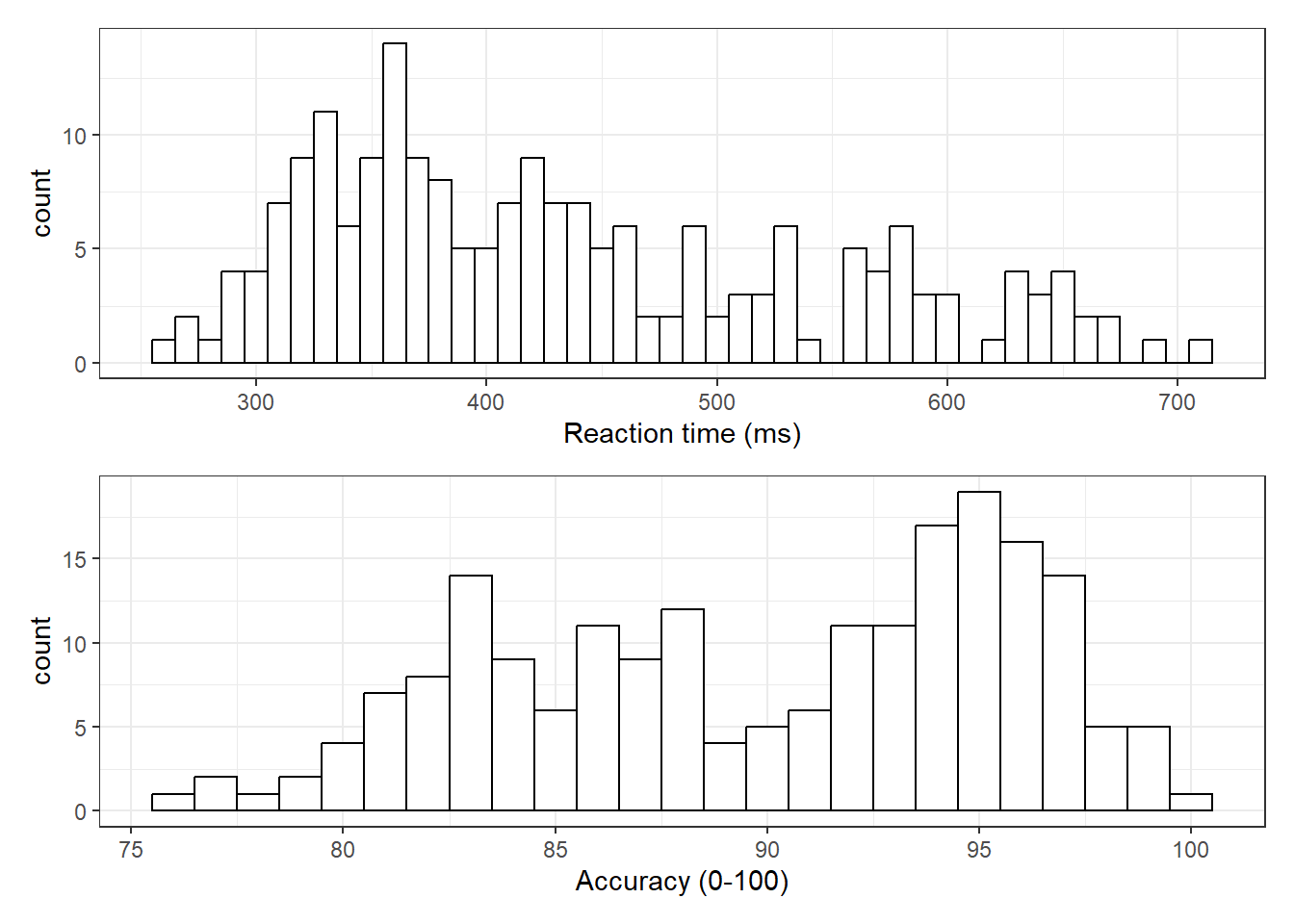Figure 4.3: Histograms showing the distribution of reaction time (top) and accuracy (bottom)

### 4.5.2 Long to wide format

Following the rule that anything that shares an axis should probably be in the same column means that we will frequently need our data in long-form when using `ggplot2`, However, there are some cases when wide format is necessary. For example, we may wish to visualise the relationship between reaction time in the word and non-word conditions. This requires that the corresponding word and non-word values for each participant be in the same row. The easiest way to achieve this in our case would simply be to use the original wide-format data as the input:

``````ggplot(dat, aes(x = rt_word, y = rt_nonword)) +
geom_point() +
geom_smooth(method = "lm")``````
``## `geom_smooth()` using formula = 'y ~ x'``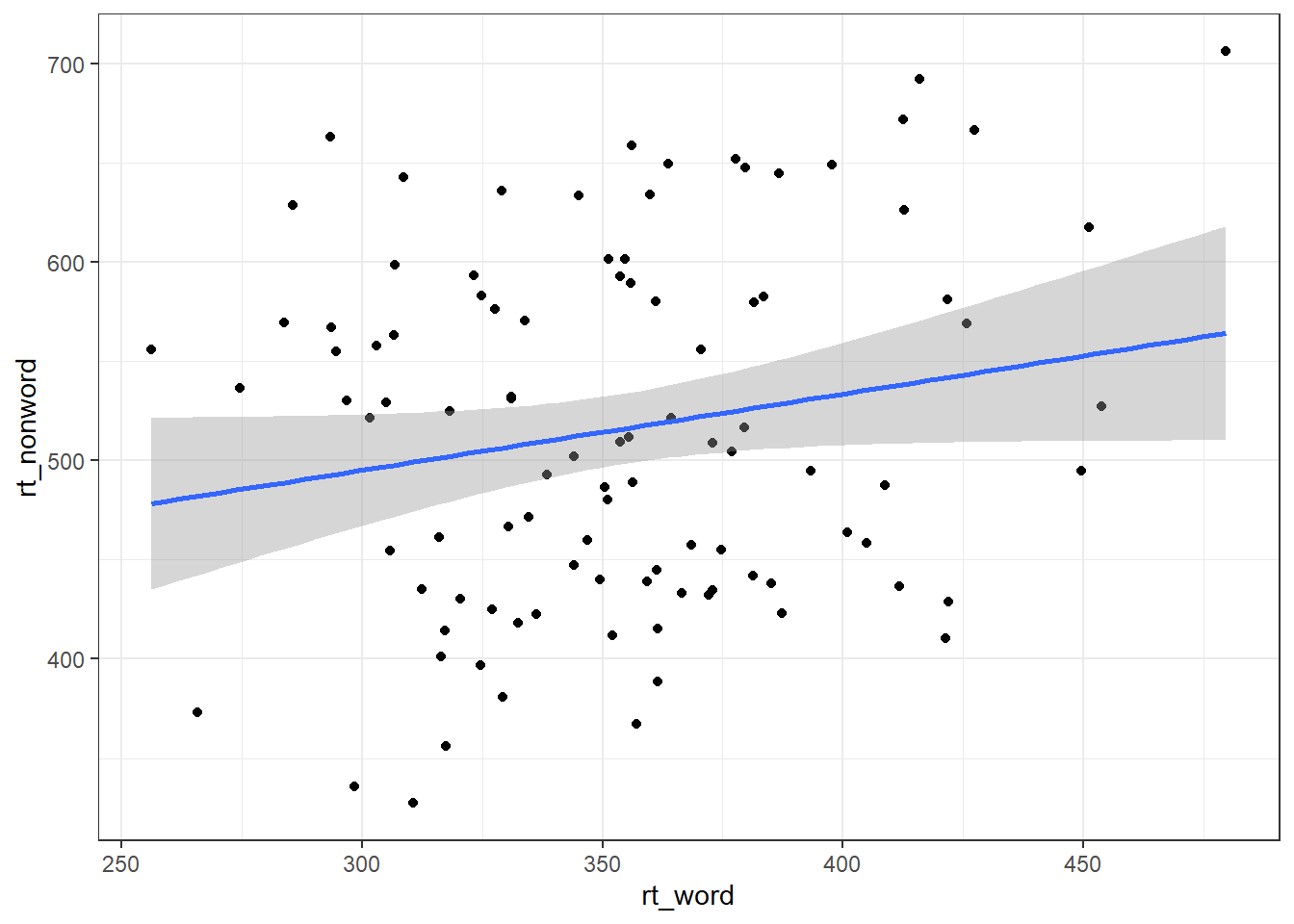However, there may also be cases when you do not have an original wide-format version and you can use the `pivot_wider()` function to transform from long to wide.

``````dat_wide <- dat_long %>%
pivot_wider(id_cols = "id",
names_from = "condition",
values_from = c(rt,acc))``````
id rt_word rt_nonword acc_word acc_nonword
S001 379.4585 516.8176 99 90
S002 312.4513 435.0404 94 82
S003 404.9407 458.5022 96 87
S004 298.3734 335.8933 92 76
S005 316.4250 401.3214 91 83
S006 357.1710 367.3355 96 78

## 4.6 Grouped plots

In the long-form dataset, because each variable has its own column, it's much easier to specify that you want to create grouped plots.

For example, we can create grouped density plots by adding `fill = condition`:

``````ggplot(dat_long, aes(x = rt, fill = condition)) +
geom_density(alpha = 0.75)``````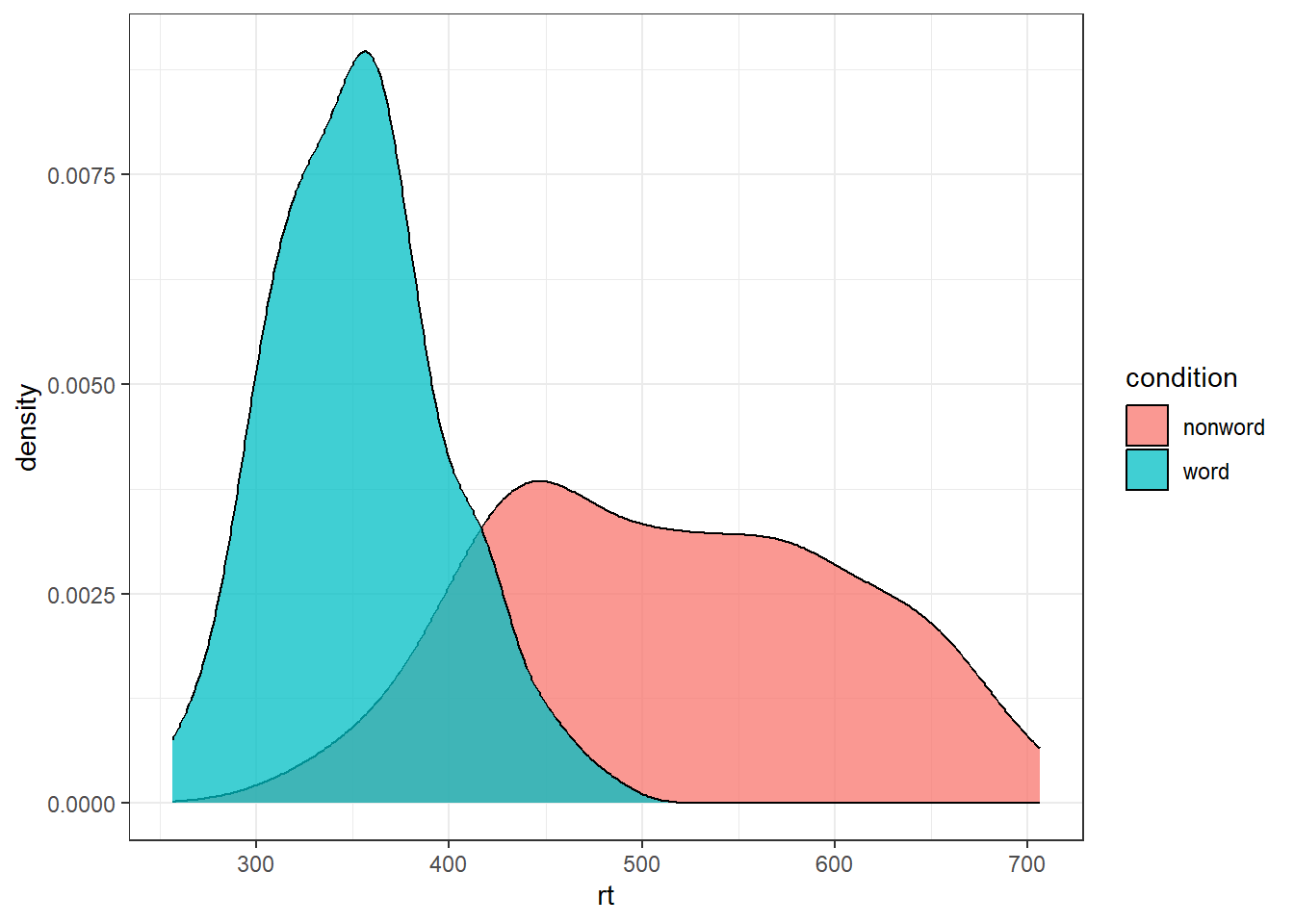And grouped scatterplots by adding `colour = condition`:

``````ggplot(dat_long, aes(x = rt, y = age, colour = condition)) +
geom_point() +
geom_smooth(method = "lm") ``````
``## `geom_smooth()` using formula = 'y ~ x'``## 4.7 Accessible colour schemes

One of the drawbacks of using `ggplot2` for visualisation is that the default colour scheme is not accessible (or visually appealing). The red and green default palette is difficult for colour-blind people to differentiate, and also does not display well in greyscale. You can specify exact custom colours for your plots, but one easy option is to use a custom colour palette. These take the same arguments as their default `scale` sister functions for updating axis names and labels, but display plots in contrasting colours that can be read by colour-blind people and that also print well in grey scale. For categorical colours, the "Set2", "Dark2" and "Paired" palettes from the `brewer` scale functions are colourblind-safe (but are hard to distinhuish in greyscale). For continuous colours, such as when colour is representing the magnitude of a correlation in a tile plot, the `viridis` scale functions provide a number of different colourblind and greyscale-safe options.

``````ggplot(dat_long, aes(x = rt, y = age, colour = condition)) +
geom_point() +
geom_smooth(method = "lm") +
scale_color_brewer(palette = "Dark2",
name = "Condition",
labels = c("Word", "Non-word"))``````
``## `geom_smooth()` using formula = 'y ~ x'``## 4.8 Visualising summary statistis

Commonly, rather than visualising distributions of raw data, researchers will wish to visualise means using a bar chart with error bars. As with SPSS and Excel, `ggplot2` requires you to calculate the summary statistics and then plot the summary. There are at least two ways to do this, in the first you make a table of summary statistics and then plot that table. The second approach is to calculate the statistics within a layer of the plot. That is the approach we will use below.

First we present code for making a bar chart. The code for bar charts is here because it is a common visualisation that is familiar to most researchers. However, we would urge you to use a visualisation that provides more transparency about the distribution of the raw data, such as violin-boxplots .

To summarise the data into means, we use `stat_summary()`. Rather than calling a `geom_*` function, we call `stat_summary()` and specify how we want to summarise the data and how we want to present that summary in our figure.

• `fun` specifies the summary function that gives us the y-value we want to plot, in this case, `mean`.

• `geom` specifies what shape or plot we want to use to display the summary. For the first layer we will specify `bar`. As with the other geom-type functions we have shown you, this part of the `stat_summary()` function is tied to the aesthetic mapping in the first line of code. The underlying statistics for a bar chart means that we must specify and IV (x-axis) as well as the DV (y-axis).

``````ggplot(dat_long, aes(x = condition, y = rt)) +
stat_summary(fun = "mean", geom = "bar")``````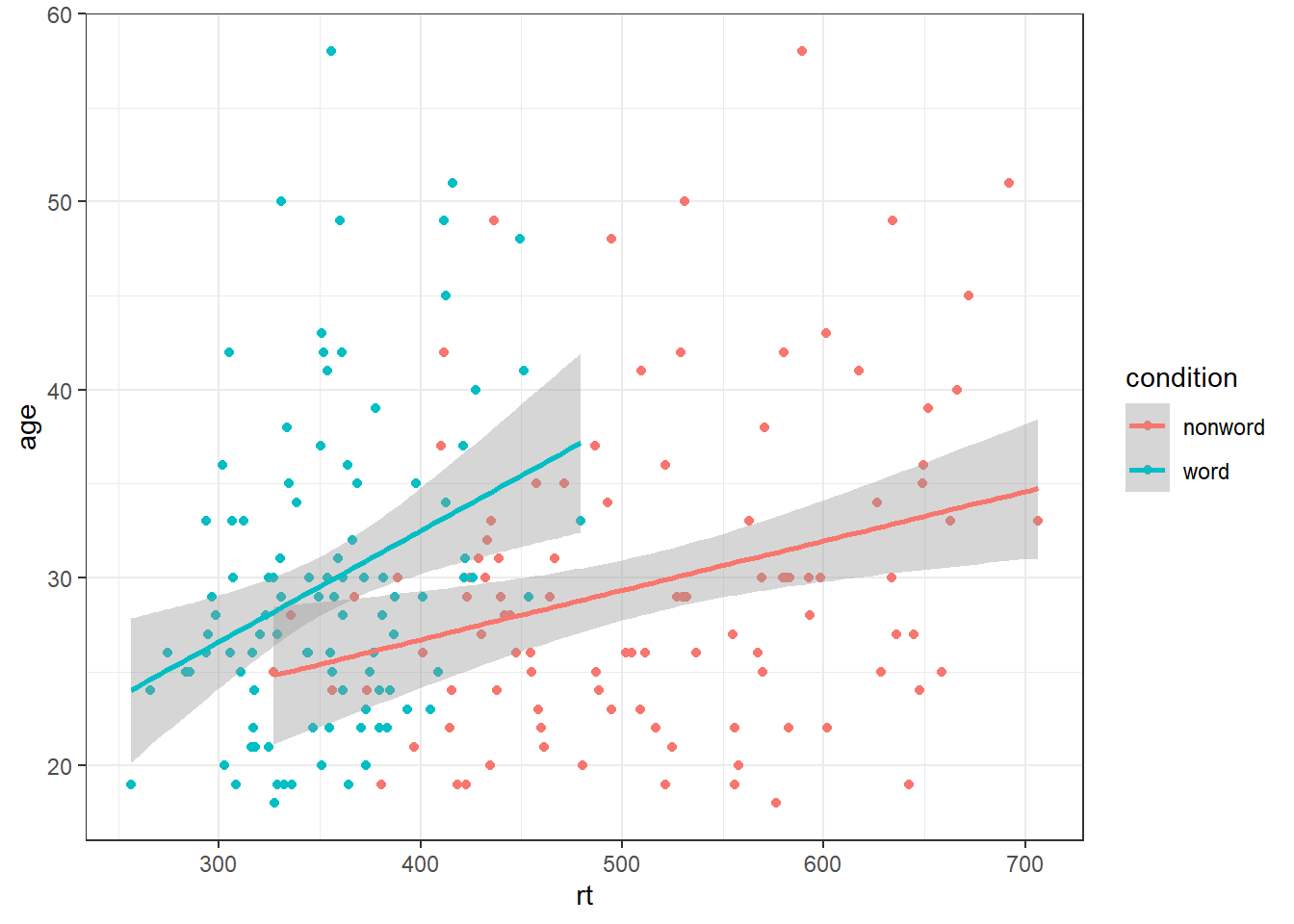To add the error bars, another layer is added with a second call to `stat_summary`. This time, the function represents the type of error bars we wish to draw, you can choose from `mean_se` for standard error, `mean_cl_normal` for confidence intervals, or `mean_sdl` for standard deviation. `width` controls the width of the error bars - try changing the value to see what happens.

• Whilst `fun` returns a single value (y) per condition, `fun.data` returns the y-values we want to plot plus their minimum and maximum values, in this case, `mean_se`
``````ggplot(dat_long, aes(x = condition, y = rt)) +
stat_summary(fun = "mean", geom = "bar") +
stat_summary(fun.data = "mean_se",
geom = "errorbar",
width = .2)``````### 4.8.1 Grouped violin-boxplots

As with previous plots, another variable can be mapped to `fill` for the violin-boxplot and we can also use `stat_summary()` to add in the mean and errorbars. However, simply adding `fill` to the mapping causes the different components of the plot to become misaligned because they have different default positions:

``````ggplot(dat_long, aes(x = condition, y= rt, fill = language)) +
geom_violin() +
geom_boxplot(width = .2,
fatten = NULL) +
stat_summary(fun = "mean",  geom = "point") +
stat_summary(fun.data = "mean_se",
geom = "errorbar",
width = .1) +
scale_fill_brewer(palette = "Dark2")``````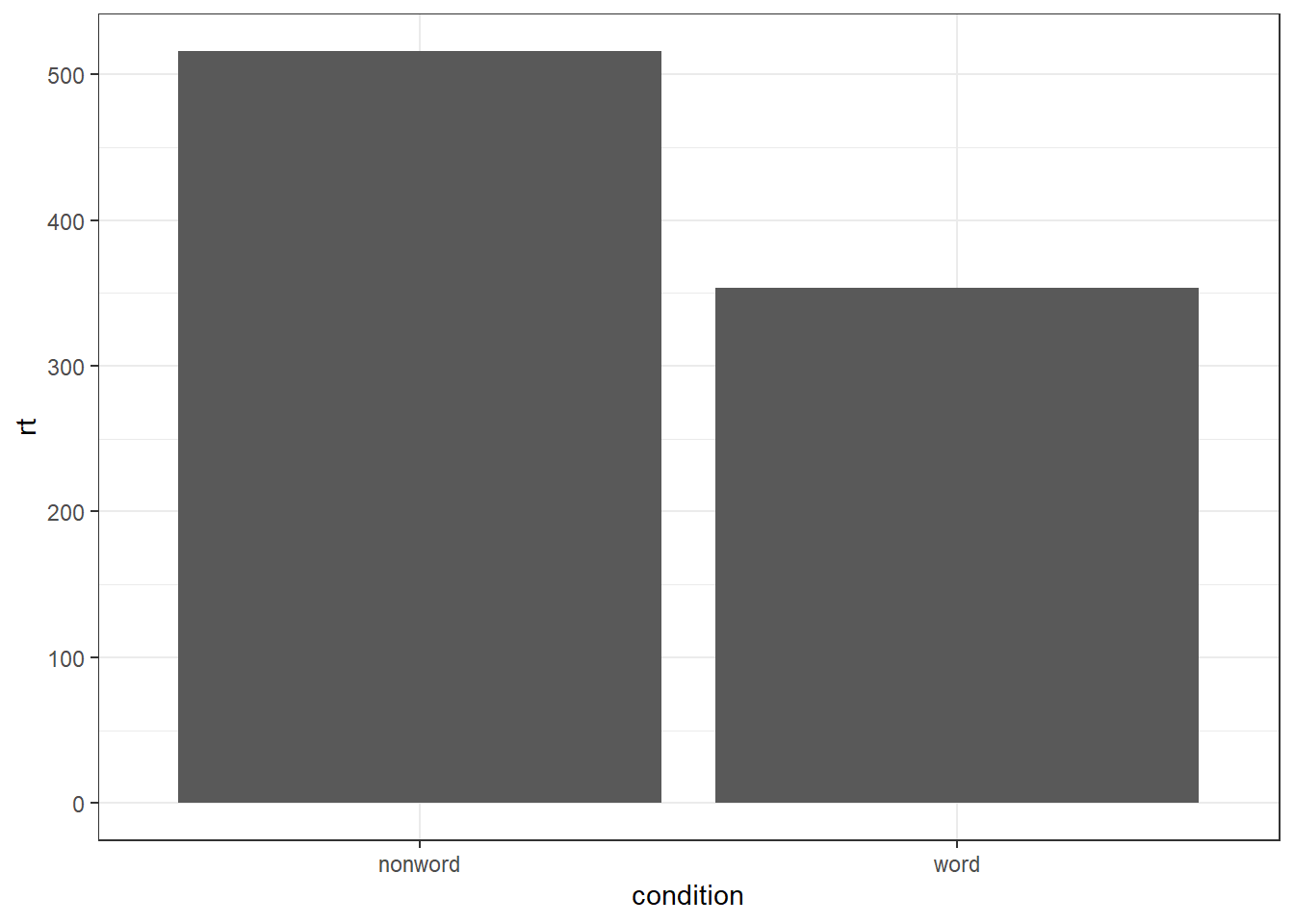To rectify this we need to adjust the argument `position` for each of the misaligned layers. `position_dodge()` instructs R to move (dodge) the position of the plot component by the specified value; finding what value looks best can sometimes take trial and error. We can also set the `alpha` values to make it easier to distinguish each layer of the plot.

``````ggplot(dat_long, aes(x = condition, y= rt, fill = language)) +
geom_violin(alpha = 0.25, position = position_dodge(0.9)) +
geom_boxplot(width = .2,
fatten = NULL,
alpha = 0.75,
position = position_dodge(0.9)) +
stat_summary(fun = "mean",
geom = "point",
position = position_dodge(0.9)) +
stat_summary(fun.data = "mean_se",
geom = "errorbar",
width = .1,
position = position_dodge(0.9)) +
scale_fill_brewer(palette = "Dark2")``````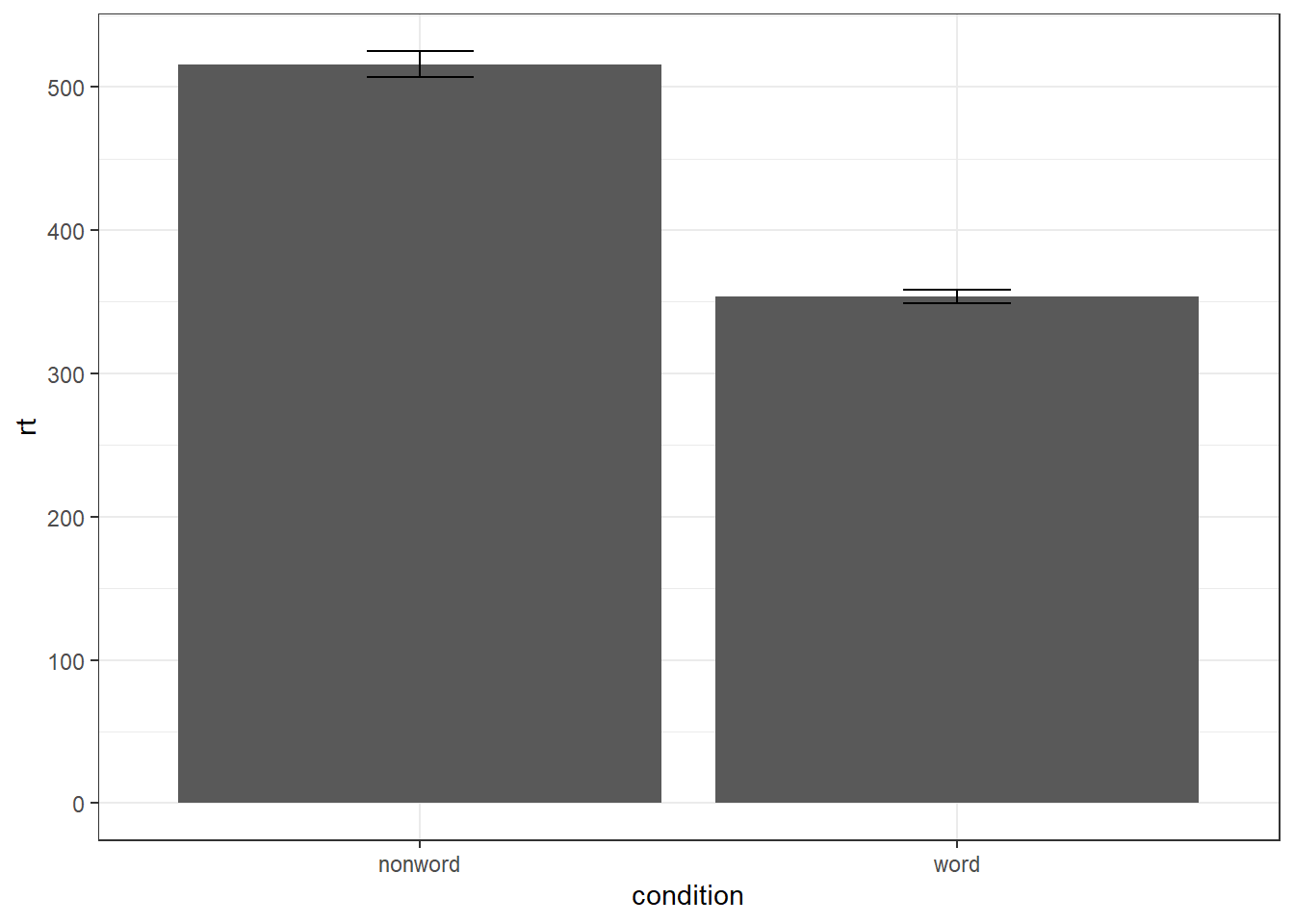### 4.8.2 Combined interaction plots

A more complex interaction plot can be produced that takes advantage of the layers to visualise not only the overall interaction, but the change across conditions for each participant.

This code is more complex than all prior code because it does not use a universal mapping of the plot aesthetics. In our code so far, the aesthetic mapping (`aes`) of the plot has been specified in the first line of code because all layers used the same mapping. However, is is also possible for each layer to use a different mapping -- we encourage you to build up the plot by running each line of code sequentially to see how it all combines.

• The first call to `ggplot()` sets up the default mappings of the plot that will be used unless otherwise specified - the `x`, `y` and `group` variable. Note the addition of `shape`, which will vary the shape of the geom according to the language variable.
• `geom_point()` overrides the default mapping by setting its own `colour` to draw the data points from each language group in a different colour. `alpha` is set to a low value to aid readability.
• Similarly, `geom_line()` overrides the default grouping variable so that a line is drawn to connect the individual data points for each participant (`group = id`) rather than each language group, and also sets the colours.
• Finally, the calls to `stat_summary()` remain largely as they were, with the exception of setting `colour = "black"` and `size = 2` so that the overall means and error bars can be more easily distinguished from the individual data points. Because they do not specify an individual mapping, they use the defaults (e.g., the lines are connected by language group). For the error bars, the lines are again made solid.
``````ggplot(dat_long, aes(x = condition, y = rt,
group = language, shape = language)) +
# adds raw data points in each condition
geom_point(aes(colour = language),alpha = .2) +
# add lines to connect each participant's data points across conditions
geom_line(aes(group = id, colour = language), alpha = .2) +
# add data points representing cell means
stat_summary(fun = "mean", geom = "point", size = 2, colour = "black") +
# add lines connecting cell means by condition
stat_summary(fun = "mean", geom = "line", colour = "black") +
# add errorbars to cell means
stat_summary(fun.data = "mean_se", geom = "errorbar",
width = .2, colour = "black") +
# change colours and theme
scale_color_brewer(palette = "Dark2") +
theme_minimal()``````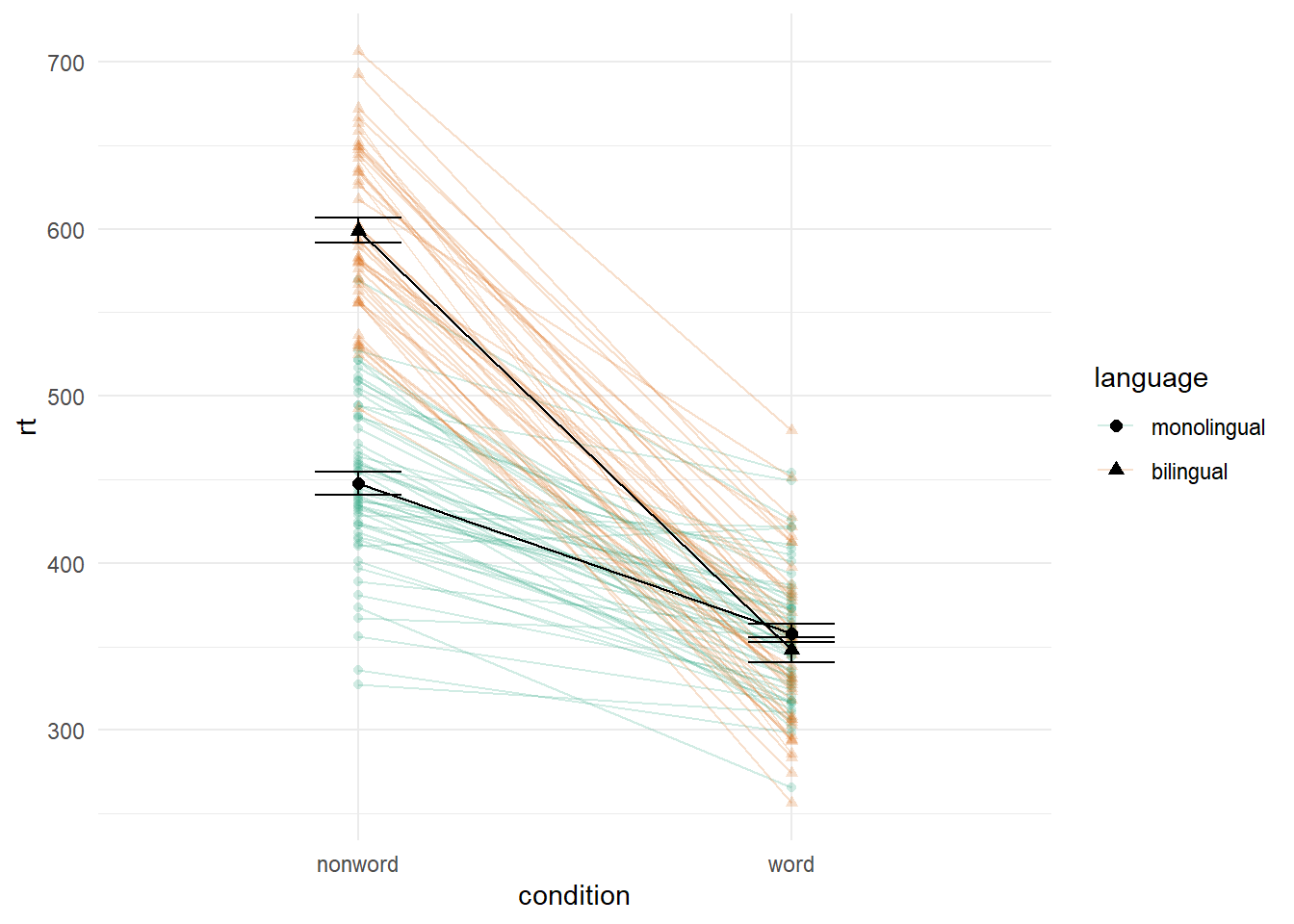Figure 4.4: Interaction plot with by-participant data.

## 4.9 Facets

There are situations in which it may be useful to create separate plots for each level of a variable using facets. This can also help with accessibility when used instead of or in addition to group colours. The below code is an adaptation of the code used to produce the grouped scatterplot in which it may be easier to see how the relationship changes when the data are not overlaid.

• Rather than using `colour = condition` to produce different colours for each level of `condition`, this variable is instead passed to `facet_wrap()`.
``````ggplot(dat_long, aes(x = rt, y = age)) +
geom_point() +
geom_smooth(method = "lm") +
facet_wrap(~condition, nrow = 2)``````
``## `geom_smooth()` using formula = 'y ~ x'``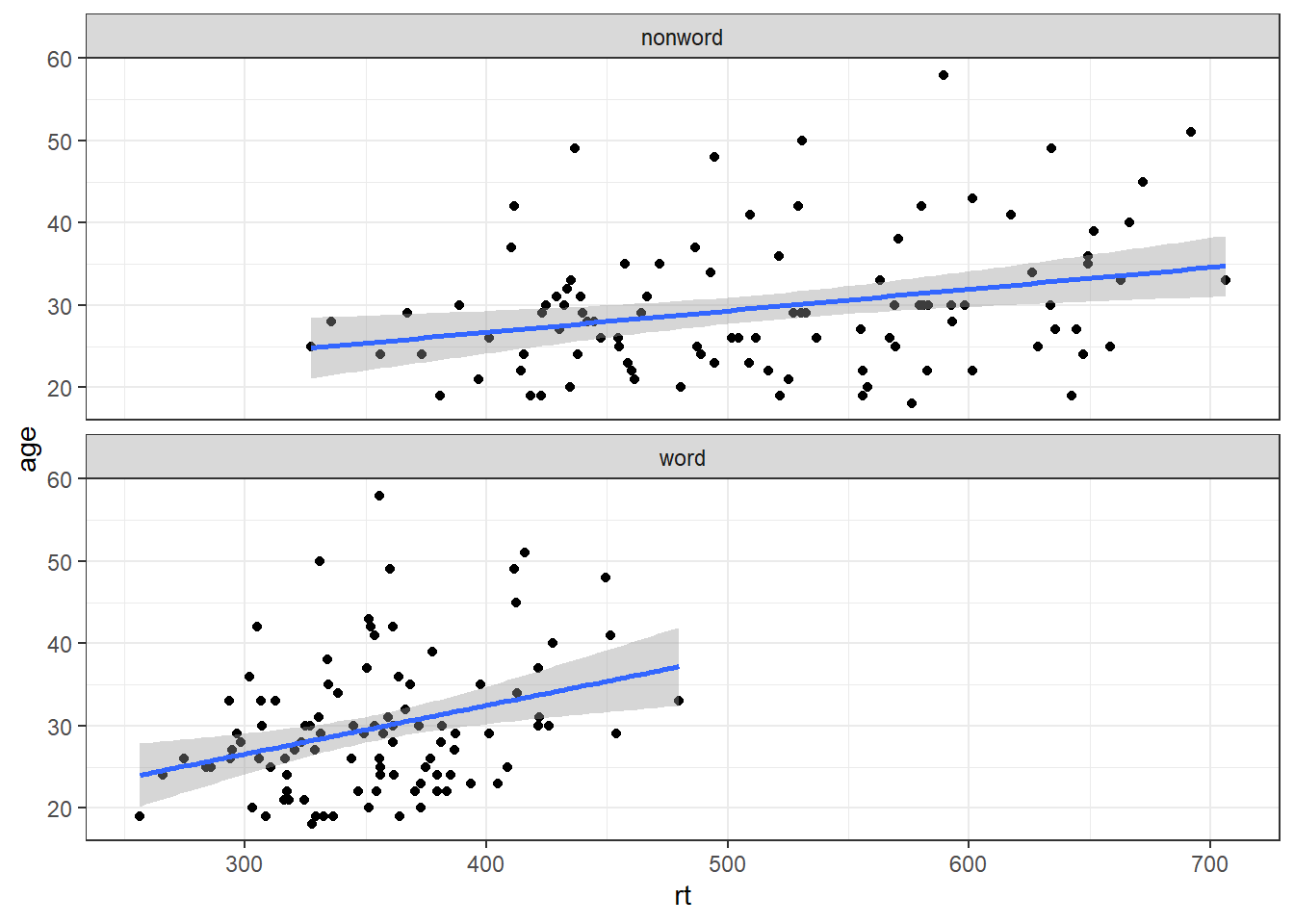Figure 4.5: Faceted scatterplot

As another example, we can use `facet_wrap()` as an alternative to the grouped violin-boxplot ( in which the variable `language` is passed to `facet_wrap()` rather than `fill`.

``````ggplot(dat_long, aes(x = condition, y= rt)) +
geom_violin() +
geom_boxplot(width = .2, fatten = NULL) +
stat_summary(fun = "mean", geom = "point") +
stat_summary(fun.data = "mean_se", geom = "errorbar", width = .1) +
facet_wrap(~language) +
theme_minimal()``````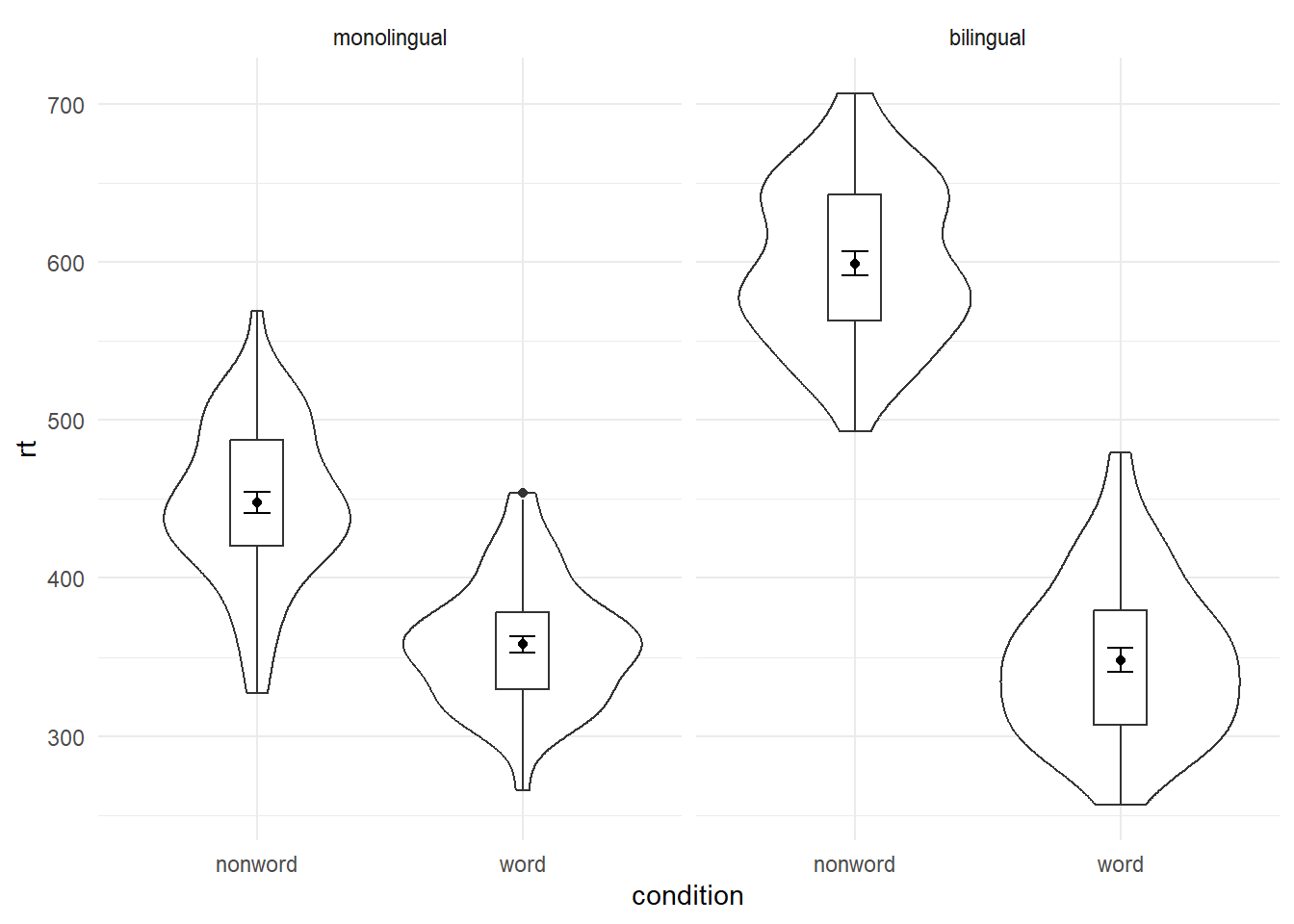Figure 4.6: Facted violin-boxplot

## 4.10 Axis labels

Previously when we edited the main axis labels we used the `scale_*` functions. These functions are useful to know because they allow you to customise many aspects of the scale, such as the breaks and limits. However, if you only need to change the main axis `name`, there is a quicker way to do so using `labs()`. The below code adds a layer to the plot that changes the axis labels for the histogram saved in `p1` and adds a title and subtitle. The title and subtitle do not conform to APA standards (more on APA formatting in the additional resources), however, for presentations and social media they can be useful.

``````ggplot(dat, aes(age)) +
geom_histogram(binwidth = 1, fill = "wheat", color = "black") +
labs(x = "Age", title = "Histogram of participant ages", subtitle = "Full sample")``````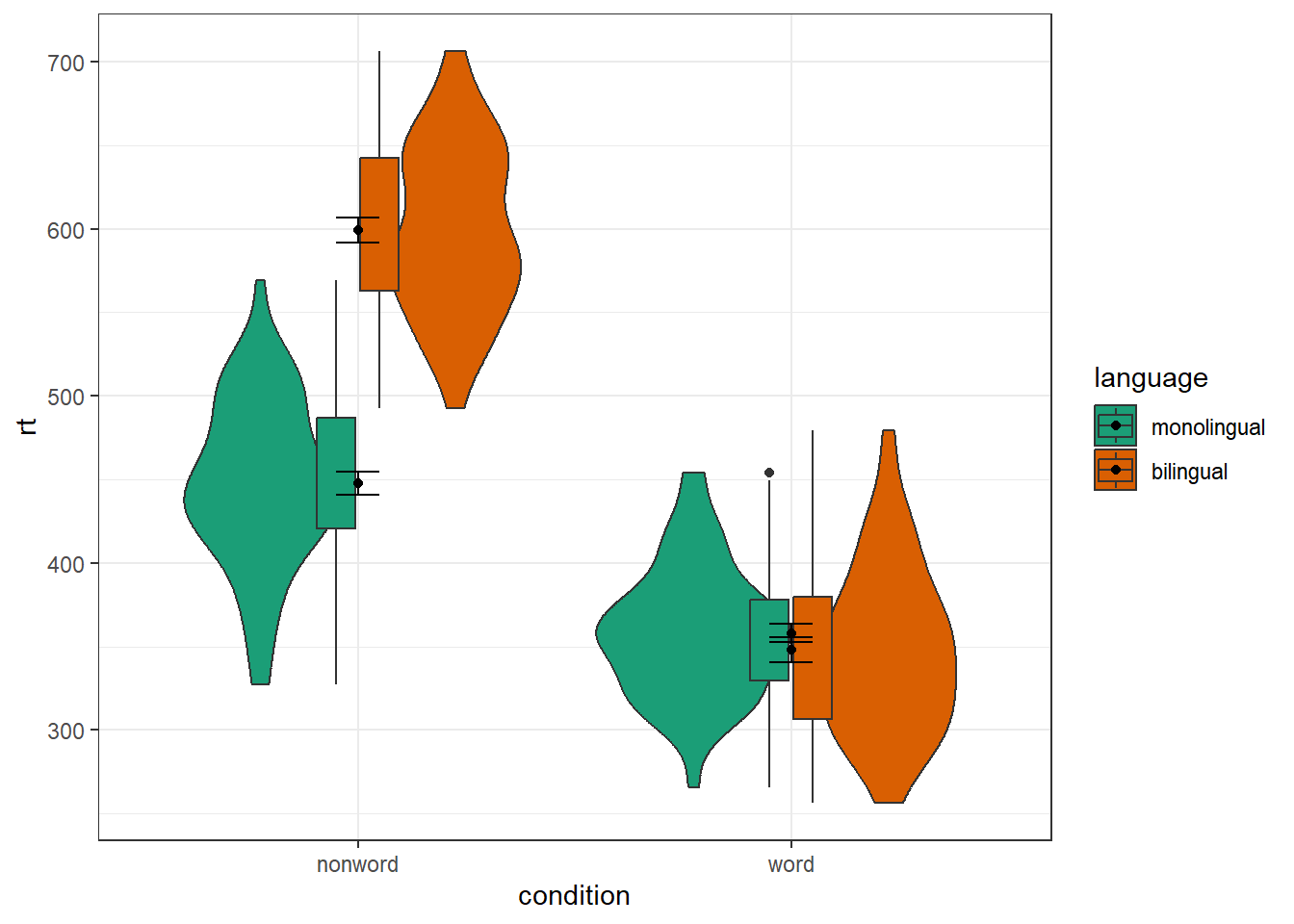You can also use `labs()` to remove axis labels, for example, try adjusting the above code to `x = NULL`.

## 4.11 Redundant aesthetics

So far when we have produced plots with colours, the colours were the only way that different levels of a variable were indicated, but it is sometimes preferable to indicate levels with both colour and other means, such as facets or x-axis categories.

The code below adds `fill = condition` to violin-boxplots. We adjust `alpha` and use the brewer colour palette to customise the colours. Specifying a `fill` variable means that by default, R produces a legend for that variable. However, the use of colour is redundant with the x-axis labels, so you can remove this legend with the `guides` function.

``````ggplot(dat_long, aes(x = condition, y= rt, fill = condition)) +
geom_violin(alpha = .4) +
geom_boxplot(width = .2, fatten = NULL, alpha = .6) +
stat_summary(fun = "mean", geom = "point") +
stat_summary(fun.data = "mean_se", geom = "errorbar", width = .1) +
theme_minimal() +
scale_fill_brewer(palette = "Dark2") +
guides(fill = "none")``````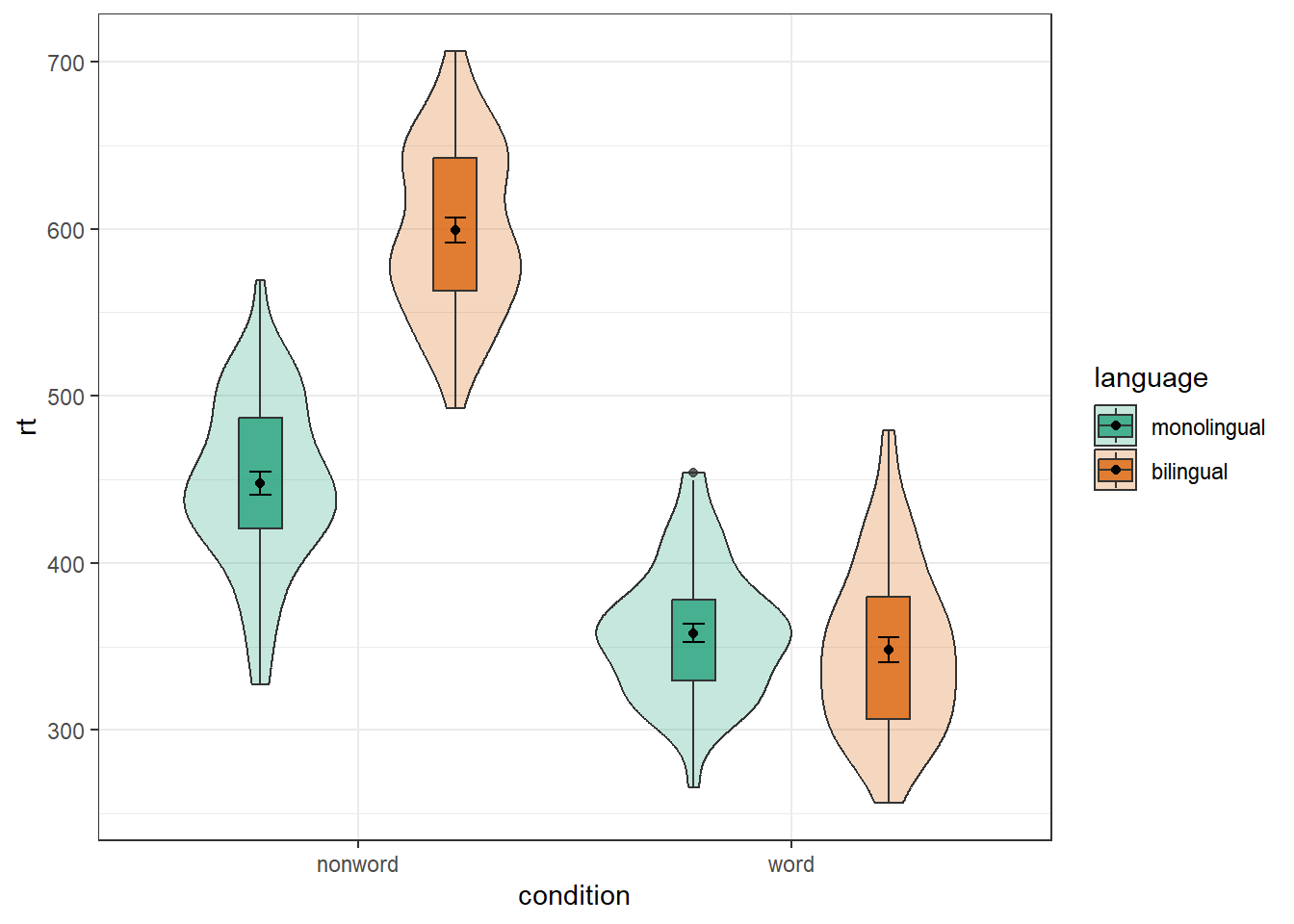For the advanced plots, we will use some custom functions: `geom_split_violin()` and `geom_flat_violin()`, which you can access through the `introdataviz` package. These functions are modified from .

``````# how to install the introdataviz package to get split and half violin plots
devtools::install_github("psyteachr/introdataviz")

# if you get the error "there is no package called "devtools" run:
# install.packages("devtools") ``````

### 4.12.1 Split-violin plots

Split-violin plots remove the redundancy of mirrored violin plots and make it easier to compare the distributions between multiple conditions.

``````ggplot(dat_long, aes(x = condition, y = rt, fill = language)) +
introdataviz::geom_split_violin(alpha = .4) +
geom_boxplot(width = .2, alpha = .6) +
stat_summary(fun.data = "mean_se", geom = "pointrange",
position = position_dodge(.175)) +
scale_x_discrete(name = "Condition", labels = c("Non-word", "Word")) +
scale_y_continuous(name = "Reaction time (ms)",
breaks = seq(200, 800, 100),
limits = c(200, 800)) +
scale_fill_brewer(palette = "Dark2", name = "Language group") +
theme_minimal() +
guides(fill = "none")``````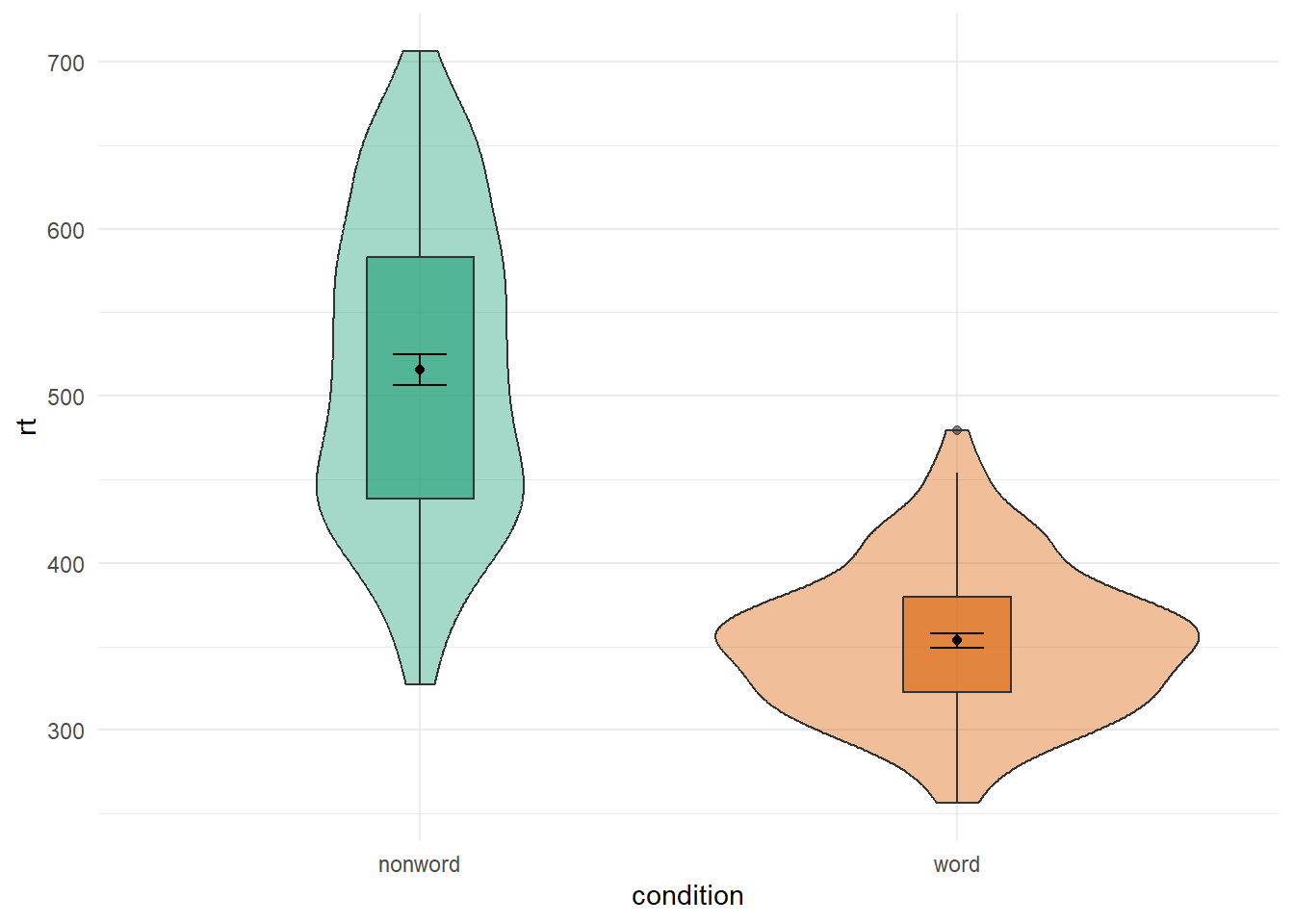## 4.13 Raincloud plots

Raincloud plots combine a density plot, boxplot, raw data points, and any desired summary statistics for a complete visualisation of the data. They are so called because the density plot plus raw data is reminiscent of a rain cloud. The point and line in the centre of each cloud represents its mean and 95% CI. The rain represents individual data points.

``````rain_height <- .1

ggplot(dat_long, aes(x = "", y = rt, fill = language)) +
# clouds
introdataviz::geom_flat_violin(alpha = 0.4,
position = position_nudge(x = rain_height+.05)) +
# rain
geom_point(aes(colour = language), size = 2, alpha = .5,
position = position_jitter(width = rain_height, height = 0)) +
# boxplots
geom_boxplot(width = rain_height, alpha = 0.4,
position = position_nudge(x = -rain_height*2)) +
# mean and SE point in the cloud
stat_summary(fun.data = mean_cl_normal, mapping = aes(color = language),
position = position_nudge(x = rain_height * 3)) +
scale_x_discrete(name = "", expand = c(rain_height*3, 0, 0, 0.7)) +
scale_y_continuous(name = "Reaction time (ms)",
breaks = seq(200, 800, 100),
limits = c(200, 800)) +
coord_flip() +
facet_wrap(~factor(condition,
levels = c("word", "nonword"),
labels = c("Word", "Non-Word")),
nrow = 2) +
# custom colours and theme
scale_fill_brewer(palette = "Dark2", name = "Language group") +
scale_colour_brewer(palette = "Dark2") +
theme_minimal() +
theme(panel.grid.major.y = element_blank(),
legend.position = c(0.8, 0.8),
legend.background = element_rect(fill = "white", color = "white")) +
guides(fill = "none")``````
``````## Warning: Using the `size` aesthietic with geom_polygon was deprecated in ggplot2 3.4.0.